Share

# Selina solutions for Concise Mathematics Class 10 ICSE chapter 20 - Cylinder, Cone and Sphere [Latest edition]

Course
Textbook page

#### Chapters## Chapter 20: Cylinder, Cone and Sphere

Exercise 20(A)Exercise 20(B)Exercise 20(C)Exercise 20(D)Exercise 20(E)Exercise 20(F)Exercise 20(G)

### Selina solutions for Concise Mathematics Class 10 ICSE Chapter 20 Cylinder, Cone and Sphere Exercise 20(A) [Pages 297 - 298]

Exercise 20(A) | Q 1.1 | Page 297

The height of a circular cylinder is 20 cm and the radius of its base is 7 cm. Find : the volume

Exercise 20(A) | Q 1.2 | Page 297

The height of a circular cylinder is 20 cm and the radius of its base is 7 cm. Find : the total surface area.

Exercise 20(A) | Q 2 | Page 297

The inner radius of a pipe is 2.1 cm. How much water can 12 m of this pipe hold?

Exercise 20(A) | Q 3.1 | Page 297

A cylinder of circumference 8 cm and length 21 cm rolls without slideing for 4 1/2 seconds at the rate of 9 complete round per second.

find, distance travelling by the cylinder in 4 1/2 second,

Exercise 20(A) | Q 3.2 | Page 297

A cylinder of circumference 8 cm and length 21 cm rolls without sliding for 4 1/2 seconds at the rate of 9 complete rounds per second. Find the area covered by the cylinder in  4 1/2 seconds.

Exercise 20(A) | Q 4 | Page 297

How many cubic meters of earth must be dug out to make a well 28 m deep and 2.8 m in diameter ? Also , find the cost of plastering its inner surface ata Rs 4.50 per sq.meter.

Exercise 20(A) | Q 5 | Page 297

What length of solid cylinder 2 cm in diameter must be taken to recast into a hollow cylinder of external diameter 20 cm, 0.25 cm thickand 15 cm long?

Exercise 20(A) | Q 6.1 | Page 297

A cylinder has a diameter of 20 cm . The area of curved surface is 100 sq. cm. Find:  the height of the cylinder correct to one decimal place.

Exercise 20(A) | Q 6.2 | Page 297

A cylinder has a diameter of 20 cm. The area of curved surface is 100 sq cm. Find: the volume of the cylinder correct to one decimal place.

Exercise 20(A) | Q 7 | Page 297

A metal pipe has a bore (inner diameter) of 5 cm. The pipe is 5 mm thick all round. Find the weight, in kilogram, of 2 metres of the pipe if 1 cm3 of the metal weights 7.7 g.

Exercise 20(A) | Q 8 | Page 297

A cylinderical container with diameter of base 42 cm contains sufficient water to submerge a rectangular soild of iron with dimesions 22cm xx14 cm 10.5cm. find the rise in level of the water the solid is submerged.

Exercise 20(A) | Q 9 | Page 297

A cylindrical container with internal radius of its base 10 cm , contains water up to  a height of 7 cm. find the area of wetted surface of the cylinder.

Exercise 20(A) | Q 10 | Page 297

Find the total surface area of an open pipe of length 50 cm, external diameter 20 cm and internal diameter 6 cm.

Exercise 20(A) | Q 11 | Page 297

The height and radius of base of a cylinder area in the ratio 3: 1. if it volume is 1029 pi cm^3; find it total surface area.

Exercise 20(A) | Q 12 | Page 297

The radius of a solid right circular cylinder increases by 20% and its height decreases by 20%. Find the percentage change in its volume.

Exercise 20(A) | Q 13.1 | Page 298

The radius of a solid right circular cylinder decreases by 20% and its height increases by 10%. Find the percentage change in its : volume

Exercise 20(A) | Q 13.2 | Page 298

The radius of a solid right circular cylinder decreases by 20% and its height increases by 10%. Find the percentage change in its : curved surface area.

Exercise 20(A) | Q 14 | Page 298

Find the minimum length in cm and correct to nearest whole number of the thin metal sheet required to make a hollow and closed cylindrical box of diameter 20 cm and height 35 cm.
Given that the width of the metal sheet is 1 m. Also, find the cost of the sheet at the rate of Rs. 56 per m.
Find the area of metal sheet required, if 10% of it is wasted in cutting, overlapping, etc.

Exercise 20(A) | Q 15 | Page 298

3080 cm3 of water is required to fill a cylindrical vessel completely and 2310 cm3 of water is required to fill it upto 5 cm below the top. Find :

(ii) height of the vessel.

(iii) wetted surface area of the vessel when it is half-filled with water.

Exercise 20(A) | Q 16.1 | Page 298

Find the volume of the largest cylinder formed when a rectangular piece of paper 44 cm by 33 cm is rolled along it : shorter side.

Exercise 20(A) | Q 16.2 | Page 298

Find the volume of the largest cylinder formed when a rectangular piece of paper 44 cm by 33 cm is rolled along it : longer side.

Exercise 20(A) | Q 17 | Page 298

A metal cube of side 11 cm is completely submerged in water contained in a cylindrical vessel with diameter 28 cm. Find the rise in the level of water.

Exercise 20(A) | Q 18 | Page 298

A circular tank of diameter 2 m is dug and the earth removed is spread uniformly all around the tank to form an embankment 2 m in width and 1.6 m in height. Find the depth of the circular tank.

Exercise 20(A) | Q 19 | Page 298

The sum of the inner and the outer curved surfaces of a hollow metallic cylinder is 1056 cm2 and the volume of material in it is 1056 cm3.  Find its internal and external radii. Given that the height of the cylinder is 21 cm.

Exercise 20(A) | Q 20 | Page 298

The difference between the outer curved surface area and the inner curved surface area of a hollow cylinder is 352 cm2. If its height is 28 cm and the volume of material in it is 704 cm3;find its external curved surface area.

Exercise 20(A) | Q 21 | Page 298

The sum of the heights and the radius of a solid cylinder is 35 cm and its total surface area is 3080 cm2, find the volume of the cylinder.

Exercise 20(A) | Q 22 | Page 298

The total surface area of a solid cylinder is 616 cm2. If the ratio between its curved surface area and total surface area is 1 : 2; find the volume of the cylinder.

Exercise 20(A) | Q 23 | Page 298

A cylindrical vessel of height 24 cm and diameter 40 cm is full of water. Find the exact number of small cylindrical bottles, each of height 10 cm and diameter 8 cm, which can be filled with this water.

Exercise 20(A) | Q 24 | Page 298

Two solid cylinders, one with diameter 60 cm and height 30 cm and the other with radius 30 cm and height 60 cm, are metled and recasted into a third solid cylinder of height 10 cm. Find the diameter of the cylinder formed.

Exercise 20(A) | Q 25 | Page 298

The total surface area of a hollow cylinder, which is open from both sides, is 3575 cm2; area of the base ring is 357.5 cm2 and height is 14 cm. Find the thickness of the cylinder.

Exercise 20(A) | Q 26 | Page 298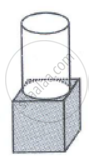The given figure shows a solid formed of a solid cube of side 40cm and a solid cylinder of radius 20 cm and height 50 cm attached to the cube as shown.
Find the volume and the total surface area of the whole solid (Take π = 3.14).

Exercise 20(A) | Q 27.1 | Page 298

Two right circular solid cylinders have radii in the ratio 3 : 5 and heights in the ratio 2 : 3, Find the ratio between their : curved surface areas.

Exercise 20(A) | Q 27.2 | Page 298

Two right circular solid cylinders have radii in the ratio 3 : 5 and heights in the ratio 2 : 3, Find the ratio between their : volumes

Exercise 20(A) | Q 28 | Page 298

A closd cylindrical tank, made of thin ironsheet , has diameter = 8.4 m and height 5.4 m. How much metal shett , to the nearest m2 , is used in making this tank , if 1/15 of the sheet actually used was wasted in making this tank , if 1/15 of the sheet actually used was wasted in making the tank ?

### Selina solutions for Concise Mathematics Class 10 ICSE Chapter 20 Cylinder, Cone and Sphere Exercise 20(B) [Page 303]

Exercise 20(B) | Q 1 | Page 303

Find the volume of a cone whose slant height is 17 cm and radius of base is 8 cm.

Exercise 20(B) | Q 2 | Page 303

The curved surface area of a cone is 12320 cm2. If the radius of its base is 56 cm, find its height.

Exercise 20(B) | Q 3 | Page 303

The circumference of the base of a 12 m high conical tent is 66 m. Find the volume of the air contained in it.

Exercise 20(B) | Q 4 | Page 303

The radius and the height of a right circular cone are in the ratio 5 : 12 and its volume is 2512 cubic cm. Find the radius and height of the cone (Take π = 3.14)

Exercise 20(B) | Q 5 | Page 303

Two right circular cone x and y are made x having three times the radius of y and y having half the volume of x. Calculate the ratio between the heights of x and y.

Exercise 20(B) | Q 6 | Page 303

The diameter of two cones are equal. If their slant heights are in the ratio 5 : 4, find the ratio of their curved surface areas.

Exercise 20(B) | Q 7 | Page 303

There are two cones. The curved surface area of one is twice that of the other. The slant height of the latter is twice that of the former find the ratio of their radii.

Exercise 20(B) | Q 8 | Page 303

A heap of wheat is in the form of a cone of diameter 16.8 m and height 3.5 m. Find its volume. How much cloth is required to just cover the heap?

Exercise 20(B) | Q 9 | Page 303

Find what length of canvas, 1.5 m in width, is required to make a conical tent 48 m in diameter and 7 m in height. Given that 10% of the canvas is used in folds and stitching’s. Also. Find the cost of the canvas at the rate of Rs. 24 per metre.

Exercise 20(B) | Q 10 | Page 303

A solid cone of height 8 cm and base radius 6 cm is melted and recast into identical cones each of height 2 cm and diameter 1 cm. Find the number of cones formed.

Exercise 20(B) | Q 11.1 | Page 303

The total surface area of a right circular cone of slant height 13 cm is 90π cm2. Calculate:  its radius in cm.

Exercise 20(B) | Q 11.2 | Page 303

The total surface area of a right circular cone of slant height 13 cm is 90π cm2. Calculate: its volume in cm3. Take π = 3.14

Exercise 20(B) | Q 12 | Page 303

The area of the base of a conical solid is 38.5 cm2 and its volume is 154 cm3. Find curved surface  area of the solid.

Exercise 20(B) | Q 13 | Page 303

A vessel, in the form of an inverted cone, is filled with water to brim. Its height is 32 cm and diameter of the base is 25.2 cm. Six equal solid cones are dropped in it, so that they are fully submerged. As a result one fourth of water is volume of each of the solid cone submerged?

Exercise 20(B) | Q 14.1 | Page 303

The volume of a conical tent is 1232 m3 and the area of the base floor is 154 m2. Calculate the: radius of the floor.

Exercise 20(B) | Q 14.2 | Page 303

The volume of a conical tent is 1232 m3 and the area of the base floor is 154 m2. Calculate the: height of the tent.

Exercise 20(B) | Q 14.3 | Page 303

The volume of a conical tent is 1232 m3 and the area of the base floor is 154 m2. Calculate the:  length of the canvas required to cover this conical tent if its width is 2 m.

### Selina solutions for Concise Mathematics Class 10 ICSE Chapter 20 Cylinder, Cone and Sphere Exercise 20(C) [Pages 305 - 307]

Exercise 20(C) | Q 1 | Page 305

The surface area of a sphere is 2464 cm^2, find its volume

Exercise 20(C) | Q 2 | Page 305

The volume of a sphere is 38808 cm^3; find its diameter and the surface area.

Exercise 20(C) | Q 3 | Page 305

A spherical ball of lead has been melted and made into identical smaller balls with radius equal to half the radius of the original one. How many such balls can be made?

Exercise 20(C) | Q 4 | Page 305

How many balls each of radius 1 cm can be made by melting a bigger ball whose diameter is 8 cm.

Exercise 20(C) | Q 5 | Page 305

Eight metallic spheres; each of radius 2 mm, are melted and cast into a single sphere. Calculate the radius of the new sphere.

Exercise 20(C) | Q 6 | Page 307

The volume of one sphere is 27 times that of another sphere. Calculate the ratio of their:

Exercise 20(C) | Q 7 | Page 307

If the number of square centimeters on the surface of a sphere is equal to the number of cubic centimeters in its volume, what is the diameter of the sphere?

Exercise 20(C) | Q 8 | Page 307

A solid metal sphere is cut through its center into 2 equal parts. If the diameter of the sphere is3 1/2 cm, find the total surface area of each part correct to two decimal places.

Exercise 20(C) | Q 9.1 | Page 306

The internal and external diameter of a hollow hemispherical vessel are 21 cm and 28 cm
respectively Find:  internal curved surface area

Exercise 20(C) | Q 9.2 | Page 306

The internal and external diameters of a hollow hemi-spherical vessel are 21 cm and 28 cm respectively. Find: external curved surface area .

Exercise 20(C) | Q 9.3 | Page 306

The internal and external diameters of a hollow hemi-spherical vessel are 21 cm and 28 cm respectively. Find: total surface area.

Exercise 20(C) | Q 9.4 | Page 306

The internal and external diameters of a hollow hemi-spherical vessel are 21 cm and 28 cm respectively. Find volume of material of the vessel.

Exercise 20(C) | Q 10 | Page 306

A solid sphere and a solid hemi-sphere have the same total surface area. Find the ratio between  their volumes.

Exercise 20(C) | Q 11 | Page 306

Metallic spheres of radii 6 cm, 8 cm and 10 cm respectively are melted and re casted into a single solid sphere. Taking π = 3.1, find the surface area of solid sphere formed.

Exercise 20(C) | Q 12.1 | Page 306

The surface area of a solid sphere is increased by 12% without changing its shape. Find the percentage increase in its: radius .

Exercise 20(C) | Q 12.2 | Page 306

The surface area of a solid sphere is increased by 21% without changing its shape. Find the percentage increase in its: volume

### Selina solutions for Concise Mathematics Class 10 ICSE Chapter 20 Cylinder, Cone and Sphere Exercise 20(D) [Page 308]

Exercise 20(D) | Q 1 | Page 308

A solid sphere of radius 15 cm is melted and recast into solid right circular cones of radius 2.5 cm and height 8 cm. Calculate the number of cones recast.

Exercise 20(D) | Q 2 | Page 308

A hollow sphere of internal and external diameter 4 cm and 8 cm respectively is melted into a cone of base diameter 8 cm. Find the height of the cone.

Exercise 20(D) | Q 3 | Page 308

The radii of the internal and external surface of a metallic spherical. It is melted and recast into a solid right circular cone of height 32 cm. Find the diameter of the base of the cone.

Exercise 20(D) | Q 4 | Page 308

Total volume of three identical cones is the same as that of a bigger cone whose height is 9 cm and diameter 40 cm. Find the radius of the base of each smaller cone, if height of each is 108 cm

Exercise 20(D) | Q 5 | Page 308

A solid rectangular block of metal 49 cm by 44 cm by 18 cm is melted and formed into a solid sphere. Calculate the radius of the sphere.

Exercise 20(D) | Q 6 | Page 308

A hemi-spherical bowl of internal radius 9 cm is full of liquid. This liquid is to be filled into conical shaped small containers each of diameter 3 cm and height 4 cm.  How many containers are necessary to empty the bowl?

Exercise 20(D) | Q 7 | Page 308

A hemispherical bowl of diameter 7.2 cm is filled completely with chocolate sauce. This sauce is poured into a inverted cone of radius 4.8 cm. Find the height of the cone is it is completely filled.

Exercise 20(D) | Q 8 | Page 308

A solid cone of radius 5 cm and height 8 cm is melted and made into small spheres of radius 0.5cm. Find the number of sphere formed.

Exercise 20(D) | Q 9.1 | Page 308

The total area of a solid metallic sphere is 1256 cm2. It is melted and recast into solid right circular cones of radius 2.5 cm and height 8 cm. Calculate: the radius of the solid sphere.

Exercise 20(D) | Q 9.2 | Page 308

The total area of a solid metallic sphere is 1256 cm2. It is melted and recast into solid right circular cones of radius 2.5 cm and height 8 cm. Calculate: the number of cones recasted [π = 3.14]

Exercise 20(D) | Q 10 | Page 308

A solid metallic cone, with radius 6 cm and height 10cm, is made of some heavy metal A. In order to reduce its weight, a conical hole is made in the cone as shown and it is completely filled with a lighter metal B. The conical hole has a diameter of cm and depth 4 cm calculate the ratio of the volume of metal A to the volume of the metal B in the solid.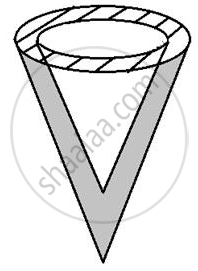Exercise 20(D) | Q 11 | Page 308

A hollow sphere of internal and external radii 6 cm and 8 cm respectively is melted and react into small cones of base radius 2 cm and height 8 cm. Find the number of cones.

Exercise 20(D) | Q 12.1 | Page 308

The surface area of a solid metallic sphere is 2464 cm2. It is melted and recast into solid right circular cones of radius 3.5 cm and height 7 cm. Calculate: the radius of the sphere   (Take π = 22/17)

Exercise 20(D) | Q 12.2 | Page 308

The surface area of a solid metallic sphere is 2464 cm2. It is melted and recast into solid right circular cones of radius 3.5 cm and height 7 cm. Calculate : the number of cones recast.  ("Take"  pi =22/7)

### Selina solutions for Concise Mathematics Class 10 ICSE Chapter 20 Cylinder, Cone and Sphere Exercise 20(E) [Pages 311 - 312]

Exercise 20(E) | Q 1 | Page 311

A cone of height 15 cm and diameter 7 cm is mounted on a hemisphere of same diameter. Determine the volume of the solid thus formed.

Exercise 20(E) | Q 2 | Page 311

A buoy is made in the form of hemisphere surmounted by a right cone whose circular base coincides with the plane surface of hemisphere. The radius of the base of the cone is 3.5 metres and its volume is two third of the hemisphere. Calculate the height of the cone and the surface area of the buoy, correct to two places of decimal

Exercise 20(E) | Q 3.1 | Page 311

From a rectangular solid of metal 42 cm by 30 cm by 20 cm, a conical cavity of diameter 14 cm
and depth 24 cm is drilled out. Find:  the surface area of remaining solid

Exercise 20(E) | Q 3.2 | Page 311

From a rectangular solid of metal 42 cm by 30 cm by 20 cm, a conical cavity of diameter 14 cm and depth 24 cm is drilled out. Find: the volume of remaining solid

Exercise 20(E) | Q 3.3 | Page 311

From a rectangular solid of metal 42 cm by 30 cm by 20 cm, a conical cavity of diameter 14 cm and depth 24 cm is drilled out. Find: the weight of the material drilled out if it weighs 7 gm per cm3.

Exercise 20(E) | Q 4 | Page 311

A cubical block of side 7 cm is surmounted by a hemisphere of the largest size. Find the surface area of the resulting solid.

Exercise 20(E) | Q 5 | Page 312

A vessel is in the form of an inverted cone its height is 8 cm and the radius of its top, which is open, is 5 cm. If is filled with water up to the rim. When lead shots each of which is a sphere of radius 0.5 cm are dropped into the vessel, one fourth of the water flows out. Find the number of lead shots sopped in the vessel.

Exercise 20(E) | Q 6 | Page 312

A hemi-spherical bowl has negligible thickness and the length of its circumference is 198 cm. Find the capacity of the bowl.

Exercise 20(E) | Q 7 | Page 312

Find the maximum volume of a cone that can be carved out of a solid hemisphere of radius r cm.

Exercise 20(E) | Q 8 | Page 312

The radii of the bases of two solid right circular cones of same height are r1 and r2 respectively. The cones area melted and recast into a solid sphere of radius R. Find the height of each cone in terms r1, r2 and R.

Exercise 20(E) | Q 9 | Page 312

A solid metallic hemisphere of diameter 28 cm is melted and recast into a number of identical solid cones, each of diameter 14 cm and height 8 cm. find the number of cones so formed.

Exercise 20(E) | Q 10 | Page 312

A cone and hemisphere have the same base and the same height. Find the ratio between their volumes.

### Selina solutions for Concise Mathematics Class 10 ICSE Chapter 20 Cylinder, Cone and Sphere Exercise 20(F) [Pages 315 - 316]

Exercise 20(F) | Q 1 | Page 315

From a solid right circular cylinder with height 10 cm and radius of the base 6 cm, a right circular cone of the same height and same base is removed. Find the volume of the remaining solid.

Exercise 20(F) | Q 2 | Page 315

From a solid cylinder whose height is 16 cm and radius is 12 cm, a conical cavity of height 8 cm and of base radius 6 cm is hollowed out. Find the volume and total surface area of the remaining  solid.

Exercise 20(F) | Q 3 | Page 315

A circus tent is cylindrical to a height of 4 m and conical above it. If its diameter is 105 m and its slant height is 80 m, Calculate the total area of canvas required. Also, find the total cost of canvas used at Rs. 15 per metre if the width is 1.5 m

Exercise 20(F) | Q 4 | Page 315

A circus tent is cylindrical to a height of 8m surmounted by a conical part. If total height of the tent is 13m and the diameter of its base is 24m; calculate:
(i) total surface area of the tent,
(ii) area of canvas, required to make this tent allowing 10% of the canvas used for folds and stitching.

Exercise 20(F) | Q 5 | Page 315

A cylindrical boiler, 2 m high, is 3.5 m in diameter. It has a hemispherical lid. Find the volume of its interior, including the part covered by the lid.

Exercise 20(F) | Q 6 | Page 315

A vessel is a hollow cylinder fitted with a hemispherical bottom of the same base. The depth of the cylindrical part is 4 2/3 m and the diameter of hemisphere is 3.5 m. Calculate the capacity and the internal surface area of the vessel.

Exercise 20(F) | Q 7 | Page 315

A wooden toy is in the shape of a cone mounted on a cylinder as shown alongside. If the height of the cone is 24 cm, the total height of the toy is 60 cm and the radius of the base of the cone = twice the radius of the base of the cylinder = 10 cm; find the total surface area of the toy.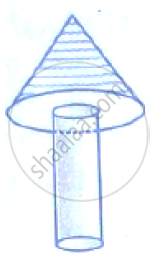Exercise 20(F) | Q 8 | Page 315

A cylindrical container with diameter of base 42 cm contains sufficient water to submerge a rectangular solid of iron with dimensions 22 cm × 14 cm × 10.5 cm. Find the rise in level of the water when the solid is submerged.

Exercise 20(F) | Q 9 | Page 315

Spherical marbles of diameter 1.4 cm are dropped into beaker containing some water and are fully submerged. The diameter of the beaker is 7 cm. Find how many marbles have been dropped in it if the water rises by 5.6 cm.

Exercise 20(F) | Q 10 | Page 316

The cross-section of a railway tunnel is a rectangle 6 m broad and 8 m high surmounted by a semi-circle as shown in the figure. The tunnel is 35 m long. Find the cost of plastering the internal surface of the tunnel (excluding the floor) at the rate of Rs. 2.25 per m^2.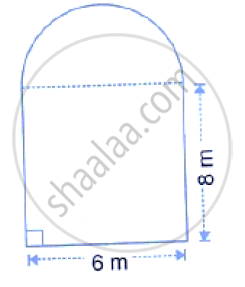Exercise 20(F) | Q 11 | Page 316

The horizontal cross-section of a water tank is in the shape of a rectangle with semi-circle at one end, as shown in the following figure. The water is 2.4 metres deep in the tank. Calculate the volume of water in the tank in gallons.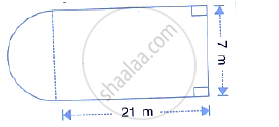Exercise 20(F) | Q 12 | Page 316

The given figure shows the cross section of a water channel consisting of a rectangle and a semicircle. Assuming that the channel is always full, find the volume of water discharged through it in one minute if water is flowing at the rate of 20 cm per second. Give your answer in cubic metres correct to one place of decimal.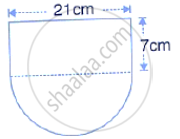Exercise 20(F) | Q 13 | Page 316

An open cylindrical vessel of internal diameter 7 cm and height 8 cm stands on a horizontal table. Inside this is placed a solid metallic right circular cone, the diameter of whose base is 3 1/2 cm and height 8 cm. Find the volume of water required to fill the vessel.
If this cone is replaced by another cone, whose height is 1 3/4 cm and the radius of whose base is 2 cm, find the drop in the water level.

Exercise 20(F) | Q 14 | Page 316

A cylindrical can, whose base is horizontal and of radius 3.5 cm, contains sufficient water so that when a sphere is placed in the can, the water just covers the sphere. Given that the sphere just fits into the can, calculate:
(i) the total surface area of the can in contact with water when the sphere is in it;
(ii) the depth of water in the can before the sphere was put into the can.

Exercise 20(F) | Q 15 | Page 316

A hollow cylinder has solid hemisphere inward at one end and on the other end it is closed with a flat circular plate. The height of water is 10 cm when flat circular surface is downward. Find the level of water, when it is inverted upside down, common diameter is 7 cm and height of the
cylinder is 20 cm.

### Selina solutions for Concise Mathematics Class 10 ICSE Chapter 20 Cylinder, Cone and Sphere Exercise 20(G) [Pages 316 - 317]

Exercise 20(G) | Q 1 | Page 316

What is the least number of solid metallic spheres, each of 6 cm diameter, that should be melted and recast to form a solid metal cone whose height is 45 cm and diameter 12 cm?

Exercise 20(G) | Q 2 | Page 316

A largest sphere is to be carved out of a right circular cylinder of radius 7 cm and height 14 cm. Find the volume of the sphere.

Exercise 20(G) | Q 3 | Page 316

A right circular cylinder having diameter 12 cm and height 15 cm is full ice-cream. The ice-cream is to be filled in cones of height 12 cm and diameter 6 cm having a hemispherical shape on the top. Find the number of such cones which can be filled with ice-cream.

Exercise 20(G) | Q 4 | Page 316

A solid is in the form of a cone standing one hemi-sphere with both their radii being equal to 8 cm and the height of cone is equal to its radius. Find, in terms of π, the volume of the solid.

Exercise 20(G) | Q 5 | Page 316

The diameter of a sphere is 6 cm, It is melted and drawn into a wire of diameter 0.2 cm. Find the length of the wire.

Exercise 20(G) | Q 6 | Page 316

Determine the ratio of the volume of a cube to that of a sphere which will exactly fit inside the cube.

Exercise 20(G) | Q 7 | Page 316

An iron pole consisting of a cylindrical portion 110 cm high and of base diameter 12 cm is surmounted by a cone 9 cm high. Find the mass of the pole, given that 1 cm3 of iron has 8 gm of mass (approx).

Exercise 20(G) | Q 8 | Page 317

In the following diagram a rectangular platform with a semi-circular end on one side is 22 metres long from one end to the other end. If the length of the half circumference is 11 metres. Find the cost of constructing the platform, 1.5 metres high at the rate of Rs. 4 per cubic metres.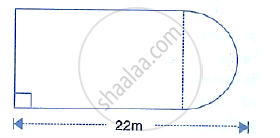Exercise 20(G) | Q 9 | Page 317

The cross-section of a tunnel is a square of side 7m surmounted by a semi circle as shown in the adjoining figure. The tunnel is 80 m long.
(1) its volume
(2) the surface area of the tunnel (excluding the floor) and
(3) its floor area.

Exercise 20(G) | Q 10 | Page 317

A cylindrical water tank of diameter 2.8 m and height 4.2 m is being fed by a pipe of diameter 7 cm through which water flows at the rater of 4 m s-1. Calculate, in minutes, the time it takes to fill the tank.

Exercise 20(G) | Q 11 | Page 317

Water flows, at 9 km per hour, through a cylindrical pipe of cross-sectional area 25 cm2. If this water is collected into a rectangular cistern of dimensions 7.5 m by 5 m by 4 m; calculate therise in level in the cistern in 1 hour 15 minutes.

Exercise 20(G) | Q 12.1 | Page 317

The given figure shows the cross-section of a cone, a cylinder and a hemisphere all with the same diameter 10 cm and the other dimensions are as shown. Calculate : the total surface area .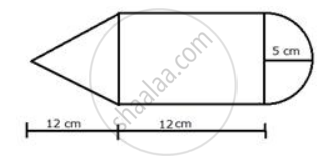Exercise 20(G) | Q 12.2 | Page 317

The given figure shows the cross-section of a cone, a cylinder and a hemisphere all with the same diameter 10 cm and the other dimensions are as shown. Calculate: the total volume of the solid.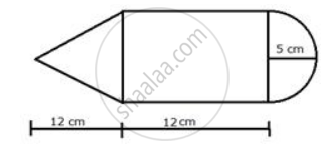Exercise 20(G) | Q 12.3 | Page 317

The given figure shows the cross-section of a cone, a cylinder and a hemisphere all with the same diameter 10 cm and the other dimensions are as shown. Calculate: the density of the material if its total weight is 1.7 kgExercise 20(G) | Q 13 | Page 317

A solid, consisting of a right circular cone, standing on a hemisphere, is placed upright, in a right circular cylinder, full of water, and touches the bottom. Find the volume of water left in the cylinder, having given that the radius of the cylinder is 3 cm and its height is 6 cm; the radius of the hemisphere is 2 cm and the height of the cone is 4 cm. Give your answer to the nearest cubic centimeter.

Exercise 20(G) | Q 14.1 | Page 317

A metal container in the form of a cylinder is surmounted by a hemisphere of the same radius. The internal height of the cylinder is 7 m and the internal radius is 3.5 m. Calculate: the total area of the internal surface, excluding the base.

Exercise 20(G) | Q 14.2 | Page 317

A metal container in the form of a cylinder is surmounted by a hemisphere of the same radius. The internal height of the cylinder is 7 m and the internal radius is 3.5 m. Calculate: the internal volume of the container in m3.

Exercise 20(G) | Q 15 | Page 317

An exhibition tent is in the form of a cylinder surmounted by a cone. The height of the tent above the ground is 85 m and height of the cylindrical part of 50 m. If the diameter of the base is 168 m, find the quantity of canvas required to make the tent. Allow 20% extra for fold and for stitching. Give your answer to the nearest m2.

Exercise 20(G) | Q 16 | Page 317

A test tube consists of a hemisphere and a cylinder of the same radius. The volume of the water required to fill the whole tube is 5159/6 ccm^3 and 4235/6 cm^3 cm3 of water is required to fill the tube to a level which is 4 cm below the top of the tune. Find the radius of the tube and the length of its  cylindrical part.

Exercise 20(G) | Q 17 | Page 317

A solid is in the form of a right circular cone mounted on a hemisphere. The diameter of the base of the cone, which exactly coincides with hemisphere, is 7 cm and its height is 8 cm. the solid is placed in a cylindrical vessel of internal radius 7 cm and height 10 cm. How much water, in cm3,  will be required to fill the vessel completely.

Exercise 20(G) | Q 18 | Page 317

Two solid spheres of radii 2 cm and 4 cm are melted and recast into a cone of height 8 cm. Find the radius of the cone so formed.

Exercise 20(G) | Q 19 | Page 317

A certain number of metallic cones, each of radius 2 cm and height 3 cm, are melted and recast into a solid sphere of radius 6 cm. Find the number of cones used.

Exercise 20(G) | Q 20 | Page 317

A conical tent is to accommodate 77 persons. Each person must have 16m3 of air to breathe. Given the radius of the tent as 7m, find the height of the tent and also its curved surface area.

## Chapter 20: Cylinder, Cone and Sphere

Exercise 20(A)Exercise 20(B)Exercise 20(C)Exercise 20(D)Exercise 20(E)Exercise 20(F)Exercise 20(G)## Selina solutions for Concise Mathematics Class 10 ICSE chapter 20 - Cylinder, Cone and Sphere

Selina solutions for Concise Mathematics Class 10 ICSE chapter 20 (Cylinder, Cone and Sphere) include all questions with solution and detail explanation. This will clear students doubts about any question and improve application skills while preparing for board exams. The detailed, step-by-step solutions will help you understand the concepts better and clear your confusions, if any. Shaalaa.com has the CISCE Concise Mathematics Class 10 ICSE solutions in a manner that help students grasp basic concepts better and faster.

Further, we at Shaalaa.com provide such solutions so that students can prepare for written exams. Selina textbook solutions can be a core help for self-study and acts as a perfect self-help guidance for students.

Concepts covered in Concise Mathematics Class 10 ICSE chapter 20 Cylinder, Cone and Sphere are Perimeter and Area of a Circle, Area and Volume of Solids - Cone, Area and Volume of Solids - Sphere, Circle - Direct Application Problems Including Inner and Outer Area, Three-dimensional Solids Right Circular Cone, Three-dimensional Solids Sphere, Volume of a Cylinder, Volume of a Combination of Solids, Surface Area of Cylinder.

Using Selina Class 10 solutions Cylinder, Cone and Sphere exercise by students are an easy way to prepare for the exams, as they involve solutions arranged chapter-wise also page wise. The questions involved in Selina Solutions are important questions that can be asked in the final exam. Maximum students of CISCE Class 10 prefer Selina Textbook Solutions to score more in exam.

Get the free view of chapter 20 Cylinder, Cone and Sphere Class 10 extra questions for Concise Mathematics Class 10 ICSE and can use Shaalaa.com to keep it handy for your exam preparation